# Modern Chemistry Chapter 3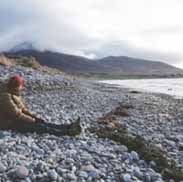Tony Foust
question

law of conservation of mass

States that matter can be neither destroyed nor created during ordinary chemical reactions or physical changes
question

law of definite proportions

The fact that a chemical compounds contains the same elements in exactly the same proportions by mass regardless of the size of the sample or source of the compound
question

law of multiple proportions

if two or more different compounds are composed of the same two elements, then the ratio of the masses of the second element combined with a certain mass of the first element is always a ratio of small whole numbers
question

atom

the smallest particle of an element that retains the chemical properties of that element
question

nucleus

very small region located near the center of an atom. made of protons and neutrons
question

Proton

positively charged particle
question

neutron

neutral particle
question

electron

negatively charged particle
question

atomic number

the number of protons in the nucleus of each atom of an element
question

isotope

atom of the same element with a different mass
question

mass number

total number of protons and neutrons in the nucleus of an isotope
question

atomic mass unit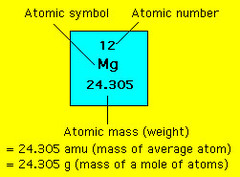exactly 1/12 the mass of a carbon-12 atom
question

average atomic mass

the weighted average of the atomic masses of naturally occurring isotopes of an element
question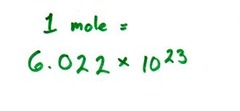6.022 x 10^23 The number of particles in exactly one mole of a pure substance
question

molar mass

the mass of one mole of a pure substance
question

Dalton

Early 1800’s, chemist who was a pioneer in discovery of the atom. Thought atoms were indivisible.
question

Thompson

English physicist accredited with the discovery and identification of the electron and isotopes.
question

Rutherford

New Zealander physicist known as the Father of Nuclear Physics. Discovered the nucleus and radioactive substances.
question

Cathode Ray Tube Experiment

This was JJ Thomson’s experiment that led to the discovery of the electron and the development of the plum pudding model of the atom. showed cathode rays were composed of negatively charged particles.
question

Plum Pudding Model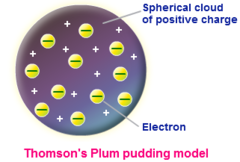model which Thomson thought electrons were randomly distributed within a positively charged cloud
question

Gold Foil Experiment

This was Ernest Rutherford’s experiment that led to the discovery of the nucleus as the small, dense, positively charged center of the atom and the development of the nuclear theory of the atom.
question

Nucleus

contains the protons and neutrons of the atom
question

Milikan

Used the oil drop experiment to determine the charge of an electron4red
question

Discovered the neutron
question

alpha particle

positively charged particles
question

ion

A charged atom that has unequal numbers of electrons and protons
question

percent abundance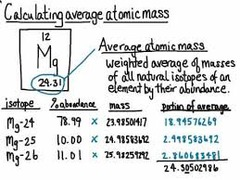the naturally occurring amount of a certain isotope of an element, out a of a 100
question

scientist who worked with and determined the proportion of particles in 1 mole
question

relative abundance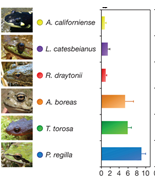the naturally occurring amount of a certain isotope of an element, out a of 1.00
question

mol

abbreviation for moles
question

Dalton’s Atomic Theory

All matter is composed of extremely small particles called atoms. Atoms of a given element are identical in size, mass, and other properties; atoms of different elements differ in size, mass, and other properties. Atoms cannot be subdivided, created, or destroyed. Atoms of different elements combine in simple whole-number ratios to form chemical compounds. In chemical reactions, atoms are combined, separated, or rearranged.
question

Modern Atomic Theory

All matter is composed of extremely small particles called atoms. A given element can have atoms with different masses; atoms of different elements differ in size, mass, and other properties. Atoms can be subdivided, but Law of Conservation of Mass holds. Atoms of different elements combine in simple whole-number ratios to form chemical compounds. In chemical reactions, atoms are combined, separated, or rearranged.
question

nuclear forces

The short-range proton-neutron, proton- proton, and neutron-neutron forces that hold the nuclear particles together
question

Atomic Number

the number of protons of each atom of that element.
question

Neutral atom

# protons = # electrons Therefore, the atomic number is also equal to the number of electrons in a neutral atom.
question

Isotopes

atoms of the same element that have different masses. all have the same number of protons and electrons, but different number of neutrons
question

Nuclide

a general term for a specific isotope of an element.
question

The mass number

is the total number of protons and neutrons that make up the nucleus of an isotope. A = #protons + #neutrons (under symbol)
question

Hyphen notation: